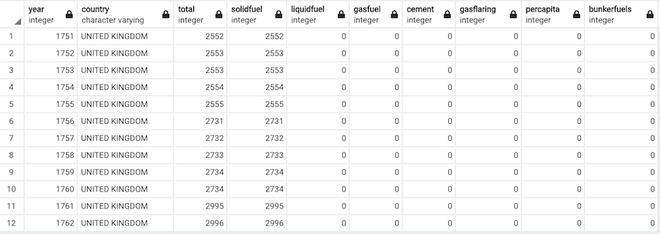Open In App

# How to insert a pandas DataFrame to an existing PostgreSQL table?

In this article, we are going to see how to insert a pandas DataFrame to an existing PostgreSQL table.

### Modules needed

• pandas: Pandas DataFrame is two-dimensional size-mutable, potentially heterogeneous tabular data structure with labeled axes (rows and columns). A Data frame is a two-dimensional data structure, i.e., data is aligned in a tabular fashion in rows and columns. Pandas DataFrame consists of three principal components, the data, rows, and columns.
• psycopg2: PostgreSQL is a powerful, open source object-relational database system. PostgreSQL runs on all major operating systems. PostgreSQL follows ACID property of DataBase system and has the support of triggers, updatable views and materialized views, foreign keys.
• sqlalchemy: SQLAlchemy is the Python SQL toolkit and Object Relational Mapper that gives application developers the full power and flexibility of SQL

we start the code by importing packages and creating a connection string of the format:

The create_engine() function takes the connection string as an argument and forms a connection to the PostgreSQL database, after connecting we create a dictionary, and further convert it into a dataframe using the method pandas.DataFrame() method.

The to_sql() method is used to insert a  pandas data frame into the Postgresql table. Finally, we execute commands using the execute() method to execute our SQL commands and fetchall() method to fetch the records.

df.to_sql(‘data’, con=conn, if_exists=’replace’, index=False)

arguments are:

• name of the table
• connection
• if_exists : if the table already exists the function we want to apply . ex: ‘append’ help us add data instead of replacing the data.
• index : True or False

Example 1:

Insert a pandas DataFrame to an existing PostgreSQL table using sqlalchemy. The create table command used to create a table in the PostgreSQL database in the following example  is:

create table data( Name varchar, Age bigint);

Code:

## Python3

 `import` `psycopg2``import` `pandas as pd``from` `sqlalchemy ``import` `create_engine`` ` ` ` `conn_string ``=` `'postgres://user:password@host/data1'`` ` `db ``=` `create_engine(conn_string)``conn ``=` `db.connect()`` ` ` ` `# our dataframe``data ``=` `{``'Name'``: [``'Tom'``, ``'dick'``, ``'harry'``],``        ``'Age'``: [``22``, ``21``, ``24``]}`` ` `# Create DataFrame``df ``=` `pd.DataFrame(data)``df.to_sql(``'data'``, con``=``conn, if_exists``=``'replace'``,``          ``index``=``False``)``conn ``=` `psycopg2.connect(conn_string``                        ``)``conn.autocommit ``=` `True``cursor ``=` `conn.cursor()`` ` `sql1 ``=` `'''select * from data;'''``cursor.execute(sql1)``for` `i ``in` `cursor.fetchall():``    ``print``(i)`` ` `# conn.commit()``conn.close()`

Output:

```('Tom', 22)
('dick', 21)
('harry', 24)```

Output in PostgreSQL:output table in PostgreSQL

Example 2:

Insert a pandas DataFrame to an existing PostgreSQL table without using sqlalchemy. As usual, we form a connection to PostgreSQL using the connect() command and execute the execute_values() method, where there’s the ‘insert’ SQL command is executed. a try-except clause is included to make sure the errors are caught if any.

The create table command used to create a table in the PostgreSQL database in the following example is :

create table fossil_fuels_c02(year int, country varchar,total int,solidfuel int, liquidfuel int,gasfuel int,cement int,gasflaring int,percapita int,bunkerfuels int);

Code:

## Python3

 `import` `psycopg2``import` `numpy as np``import` `psycopg2.extras as extras``import` `pandas as pd`` ` ` ` `def` `execute_values(conn, df, table):`` ` `    ``tuples ``=` `[``tuple``(x) ``for` `x ``in` `df.to_numpy()]`` ` `    ``cols ``=` `','``.join(``list``(df.columns))``    ``# SQL query to execute``    ``query ``=` `"INSERT INTO %s(%s) VALUES %%s"` `%` `(table, cols)``    ``cursor ``=` `conn.cursor()``    ``try``:``        ``extras.execute_values(cursor, query, tuples)``        ``conn.commit()``    ``except` `(Exception, psycopg2.DatabaseError) as error:``        ``print``(``"Error: %s"` `%` `error)``        ``conn.rollback()``        ``cursor.close()``        ``return` `1``    ``print``(``"the dataframe is inserted"``)``    ``cursor.close()`` ` ` ` `conn ``=` `psycopg2.connect(``    ``database``=``"ENVIRONMENT_DATABASE"``, user``=``'postgres'``, password``=``'pass'``, host``=``'127.0.0.1'``, port``=``'5432'``)`` ` `df ``=` `pd.read_csv(``'fossilfuels.csv'``)`` ` `execute_values(conn, df, ``'fossil_fuels_c02'``)`

Output:

`the dataframe is inserted`after inserting the dataFrame